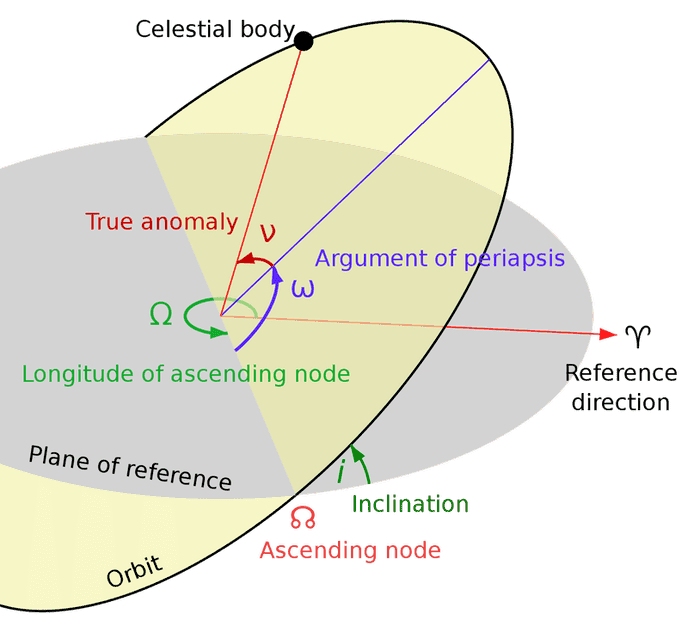# How to find RAAN and argument of perigee for a satellite?

• I
• yzma

#### yzma

I have some values and by using them I need to find the RAAN and argument of perigee of a satellite. I have the period, inclination, apogee and perigee (as values not vectors), found semi-major axis and the eccentricity, but facing difficulty on how to proceed on calculating RAAN and the argument of perigee!
any help will be greatly appreciated

For the RAAN, you'll need to take the reference direction into account (First Point of Aries), and measure the angle from that to the ascending node in an eastward direction.

https://en.wikipedia.org/wiki/Longitude_of_the_ascending_node

For the argument of perigee, measure the angle from the ascending node to the perigee.

https://en.wikipedia.org/wiki/Argument_of_periapsis•berkeman